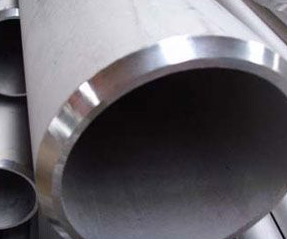Engineering ToolBox - Resources, Tools and Basic Information for Engineering and Design of Technical Applications!

# Cylinders and Pipes - Conductive Heat Losses

## Conductive heat losses through cylinder or pipe walls### Uninsulated Cylinder or Pipe

Conductive heat loss through the wall of a cylinder or pipe can be expressed as

Q = 2 π L (ti - to) / [ln(r/ ri) / k]                              (1)

where

Q = heat transfer from cylinder or pipe (W, Btu/hr)

k = thermal conductivity of piping material (W/mK or W/m oC, Btu/(hr oF ft2/ft))

L = length of cylinder or pipe (m, ft)

π = pi = 3.14...

to = temperature outside pipe or cylinder (K or oC, oF)

ti = temperature inside pipe or cylinder (K or oC, oF)

ln = the natural logarithm

ro = cylinder or pipe outside radius (m, ft)

ri = cylinder or pipe inside radius (m, ft)

### Insulated Cylinder or Pipe

Conductive heat loss through an insulated cylinder or pipe can be expressed as

Q = 2 π L (ti - to) / [(ln(r/ ri) / k) + (ln(rs / ro) / ks)]                          (2)

where

rs = outside radius of insulation (m, ft)

ks = thermal conductivity of insulation material (W/mK or W/m oC, Btu/(hr oF ft2/ft))

Equation 2 with inside convective heat resistance can be expressed as

Q = 2 π L (ti - to) / [1 / (hc ri ) + (ln(r/ ri) / k) + (ln(rs / ro) / ks)]                          (3)

where

hc = convective heat transfer coefficient (W/m2K)

## Engineering ToolBox - SketchUp Extension - Online 3D modeling!

Add standard and customized parametric components - like flange beams, lumbers, piping, stairs and more - to your Sketchup model with the Engineering ToolBox - SketchUp Extension - enabled for use with the amazing, fun and free SketchUp Make and SketchUp Pro .Add the Engineering ToolBox extension to your SketchUp from the SketchUp Pro Sketchup Extension Warehouse!

Translate

## Privacy

We don't collect information from our users. Only emails and answers are saved in our archive. Cookies are only used in the browser to improve user experience.

Some of our calculators and applications let you save application data to your local computer. These applications will - due to browser restrictions - send data between your browser and our server. We don't save this data.

## Citation

• Engineering ToolBox, (2009). Cylinders and Pipes - Conductive Heat Losses. [online] Available at: https://www.engineeringtoolbox.com/conductive-heat-loss-cylinder-pipe-d_1487.html [Accessed Day Mo. Year].

Modify access date.

. .

#### Scientific Online Calculator10 15